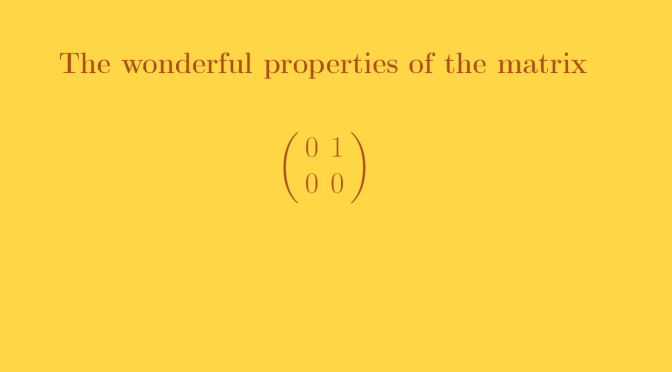One matrix having several interesting properties

We consider a vector space $$V$$ of dimension $$2$$ over a field $$\mathbb{K}$$. The matrix:
$A=\left( \begin{array}{cc} 0 & 1 \\ 0 & 0 \end{array} \right)$ has several wonderful properties!

Only zero as eigenvalue, but minimal polynomial of degree $$2$$

Zero is the only eigenvalue. The corresponding characteristic space is $$\mathbb{K} . e_1$$ where $$(e_1,e_2)$$ is the standard basis. The minimal polynomial of $$A$$ is $$\mu_A(X)=X^2$$.

$$A$$ is not diagonalizable

As zero is the only eigenvalue of $$A$$, if $$A$$ was diagonalizable, $$A$$ would be similar to the null matrix. That cannot be as the null matrix commutes with all matrices.

$$A$$ is not divisible

Suppose that $$A=B^n$$ where $$n \ge 2$$ is an integer and $$B$$ is a 2×2 matrix. As $$A^2=0$$ we have $$B^{2n}=0$$. Hence $$B$$ minimal polynomial $$\mu_B$$ divides $$X^{2n}$$. The degree of $$\mu_B$$ is less or equal to $$2$$. Therefore $$\mu_B=X$$ or $$\mu_B=X^2$$. $$\mu_B=X$$ is impossible as $$B$$ cannot be the null matrix. If $$\mu_B=X^2$$, $$B$$ would be similar to $$A$$. For the proof, consider a vector $$u$$ such that $$B.u \neq 0$$. $$(B.u,u)$$ is a base in which the matrix of $$B$$ is $$A$$. This leads to the contradiction $$0=B^2 \cdot B^{n-2}=A \neq 0$$. Finally, $$A$$ is not divisible.

$$A$$ is nilpotent but not null

We have $$A^2=0$$ while $$A \neq 0$$. Therefore $$A$$ is nilpotent.

$$A$$ can be used to prove non commutativity of matrices

Indeed we have:
$\left( \begin{array}{cc} 0 & 1 \\ 0 & 0 \end{array} \right)= \left( \begin{array}{cc} 1 & 1 \\ 0 & 0 \end{array} \right)A \neq A \left( \begin{array}{cc} 1 & 1 \\ 0 & 0 \end{array} \right)= \left( \begin{array}{cc} 0 & 0 \\ 0 & 0 \end{array} \right)$